# How many work weeks left to or passed from the specific date in Excel

Excel
When you plan a project, you can need to calculate a number of workdays or full workweeks between the start and end dates, or how many workdays or workweeks left/passed. This tip is about an easy and fast way of calculation of full workweeks for weeks from Monday to Sunday.

You easily can change these formulas to calculate the number of workweeks for weeks from Sunday to Saturday or any other workweek.

For workweeks from Monday to Sunday, do the following:

1.   To calculate a number of full workweeks between two dates, do the following:

1.1.   Calculate the next Monday from the start date:

= <start date> + 7 – WEEKDAY (<start date> + 7 - <day>)

where:

• the formula WEEKDAY () returns a number between 1 and 7 that corresponds to a particular day of the week. With default settings, 1 = Sunday, 2 = Monday, 3 = Tuesday, 4 = Wednesday, 5 = Thursday, 6 = Friday, and 7 = Saturday,
• day is the day of week that you want to find, to find next Monday, it should be 2.

For example, to calculate next Monday from the 1st of June 2018 (it is Friday):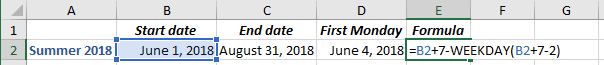1.2.   Calculate the Sunday before the end date:

= <end date> - WEEKDAY (<end date> - <day>)

For example, to calculate the Sunday before the 31st of August 2018 (it is Friday):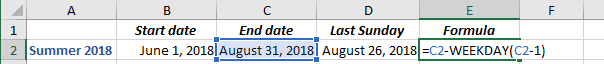1.3.   Calculate the difference between calculated dates and then divide the number of days to 7. It is necessary to return only the integer portion of the division result and discard the remainder of the division result, so, use the QUOTIENT function:

= QUOTIENT (numerator, denominator)

where:

• numerator is a dividend, a number to be divided,
• denominator is a divisor, a number to divide by.

For this example:

= QUOTIENT (<last Sunday> - <first Monday>, 7)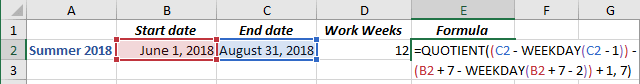2.   If you need to calculate a number of complete work weeks passed from some date, use the formula:

= QUOTIENT (TODAY () - <first Monday>, 7)

Where TODAY () calculates the current date.

For example, for the 1st of August 2018: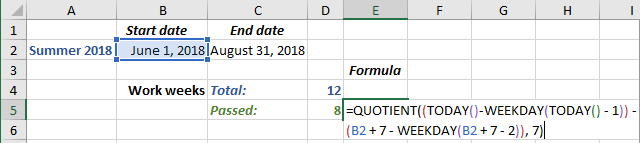3.   If you need to calculate a number of complete work weeks left to some date, use the formula:

= QUOTIENT (<last Sunday> - TODAY (), 7)

For example, for the 1st of August 2018: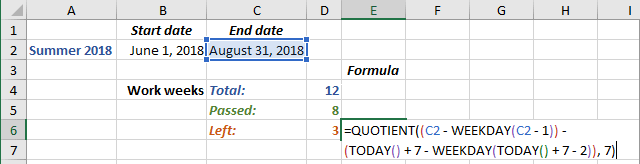So, summer 2018 contains 12 work weeks, from June 1st to August 1st there are 8 work weeks, from August 1st to August 31st there are 3 workweeks. The workweek from August 1st to August 3rd is not a full workweek, and, thus, was not counted.

If you have any questions or suggestions, please feel free to ask OfficeToolTips team.